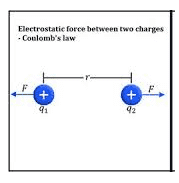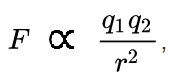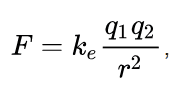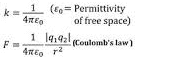# What is an electric charge? Coulombs Law of electrical charge

## What is an electric charge?

Electricity is very important aspects of our lives. It is most commonly used in domestic purpose like our home lights, computer, television etc. it deals with the study of electric charges. We know that the basic building of the matters are atoms and all the atoms are combined together which creates molecules. Naturally, the atom contains three basic elements; one is electron which contains the negative charge, the second one is proton which is responsible for positive charges and the third one is neutrons which do not have any charge particles (neither +ve charge nor –ve charge). Here atoms are electrically balanced due to both electron and proton contains equal in their quantity which means proton number is equal to electron numbers.

The charge of one electron particle is equal to 1.602 x 10 ^-19 Columb the charging unit is denoted by C. This is discovered by French physicist Charles Coulomb.

Get More Electrical Concept And Interview Questions By Using This Link

## Charges in electrically:

Electric charge is the physical property of matter (anything) that causes it to experience a force when placed in an electromagnetic field. There are two types of electric charges; positive and negative (commonly carried by protons and electrons respectively).

Also Know About:   On Delay Timer | Off Delay Timer Working Principle

Like charges (same charges) repel and unlike charges attract each other. An object with an absence of net charge is referred to as neutral. Early knowledge of how charged substances interact is now called classical electrodynamics and is still accurate for problems that don’t require consideration of quantum effects.

Get More Electrical Concept And Interview Questions By Using This Link

We know that the basic building of the matters are atoms and all the atoms are combined together which creates molecules. Naturally, the atom contains three basic elements; one is electron which contains the negative charge, the second one is proton which is responsible for positive charges and the third one is neutrons which do not have any charge particles (neither +ve charge nor –ve charge). Here atoms are electrically balanced due to both electron and proton contains equal in their quantity which means proton number is equal to electron numbers.

The charge of one electron particle is equal to 1.602 x 10 ^-19 Columb the charging unit is noted by C. This is discovered by French physicist Charles Coulomb.

Also Know About:   What is Meant by Open Circuit

The charges of the charge of the proton are equal and opposite to that of the electron, both charges are constant.

The electron revolves around the nucleus in specified orbits. The electron can be made to move from one atom to another. Here due to the electron movement, the electricity creates and also Electric charges create an electric field if they are moving they also generate a magnetic field. The combination of the electric and magnetic field is called the electromagnetic field, and its interaction with charges is the source of the electromagnetic force, which is one of the four fundamental forces in physics.

Note: The study of charged particles, and how their interactions are mediated by photons, is called quantum electrodynamics.

### Unit of Electric charge:

The SI of electric charge is the coulomb (C) for both +ve and negative charges.

The symbol Q often denotes charge.

Content has Taken under Creative common license 3.0

## Coulombs Law of electrical charge:It is used to find out the force between the two charges. Also, we can call it as Coulomb’s law, or Coulomb’s inverse-square law is a law of physics that describes force interacting between static electrically charged particles. Consider two positive charges q1 and q2, separated by a distance r. the force interaction between the charges is directionally proportional to the magnitude of charges and the inversely proportional to the square of the distance between the two charges. It is mathematically expressed as…

Also Know About:   What is Electric Field, Electric Field Intensity, Electric Field Densitywhere ke is Coulomb’s constant (ke = 8.9875×10^9 N m2 C−2), q1 and q2 are the signed magnitudes of the charges, and the scalar r is the distance between the charges. The force F of the interaction between the charges is attractive if the charges have opposite signs (i.e., F is negative) and repulsive if like-signed (i.e., F is positive).

Here the q1 and q2 is expressed by Coulombs.

r is in meter

Also, the above equation is written asGet More Electrical Concept And Interview Questions By Using This Link

Here 4 * pi is the proportionality factor, € is permeability of air.

### Example:

Find the force of interaction between the charges spaced 10 cm apart in air €=1. The charges are

8 x 10 ^-4 C and 6 x 10^-6 C respectively. Find the force of interaction?

Given Data:

r=10cm, q1= 8 x 10 ^-4 C, q2= 6x 10 ^-6 C, € =1

Solution:

F = 9 x 10^9 x 8 x 10 ^-4 x 6x 10 ^-6/ (1 x (10 x 10^2)^2)

F= 8640 N